Courses

# MCQs Question & Solutions (Competition Preparation)- Basic Concept of Chemistry, Part- 1 Class 11 Notes | EduRev

## JEE : MCQs Question & Solutions (Competition Preparation)- Basic Concept of Chemistry, Part- 1 Class 11 Notes | EduRev

The document MCQs Question & Solutions (Competition Preparation)- Basic Concept of Chemistry, Part- 1 Class 11 Notes | EduRev is a part of the JEE Course Chemistry for JEE.
All you need of JEE at this link: JEE

Q.1. The number of significant figures in π are:
(a) One
(b) Two
(c) Three
(d) Infinite.

Ans. (d)
Solution.
Significant figures in π are infinite it is irrational number (22/7) there are infinite digit in π = 3.1428571432479 (more than trillion digits in π)

Q.2. Given the numbers 786, 0.786 and 0.0786. The number of significant figures for the three numbers is:
(a) 3, 4 and 5 respectively
(b) 3, 3 and 3 respectively
(c) 3, 3 and 4 respectively
(d) 3, 4 and 4 respectively

Ans. (b)
Solution.
If zero at the left hand side of non zero digit it is not a significant figures as
0.004321 = 4
0.00231 = 3
786 = 3 (s.f), 0.786 = 3 (s.f), 0.0786 = 3 (s.f)

Q.3. In which of the following numbers all zeros are significant?
(a) 0.0005
(b) 0.0500
(c) 50.000
(d) 0.0050

Ans. (c)
Solution.
(a) 0.0005 = no any zero consider in significant number
(b) 0.0500 = only two zero consider in significant number
(c) 50.000 = all zero consider in significant number
(d) 0.0050 = only one zero consider in significant number

Q.4. 108 ÷ 7.2 = 14.583. The correct answer to this problem in proper number of significant digits is
(a) 15
(b) 14.58
(c) 14.5
(d) None of these

Ans. (a)
Solution.
In the multiplication & division significant figures are adjusted according to the least significant figure

Q.5. 14.90 + 0.0070 + 1.0 + 0.081 = 15.9880. The sum to proper number of significant digit is
(a) 15.9
(b) 16.0
(c) 15.99
(d) 16

Ans. (b)
Solution.
In the result of addition & substracting least significant figure are adjusted after the decimal place.
3.21 ( 3. Significant figure – 2 decimal places)
14.90 + 0.0070 + 1.0 + 0.081 = 15.9880
Answer will be reported after decimal place one digit
15.9880 → Rounding off upto 3 digit
More than 5 ↑ so, previous digit increased by one
= 16.0

Q.6. Which is larger quantity?
(a) Mega
(b) Femto
(c) Milli
(d) Giga

Ans. (d)
Solution.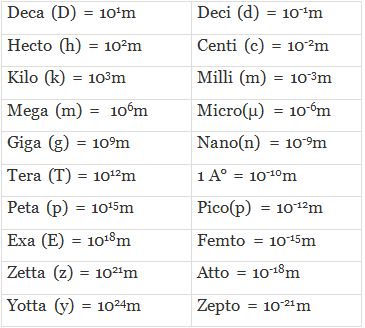Q.7. The scientific notation of 0.0000000540 is
(a) 5.40 × 10-7
(b) 5.40 × 10-8
(c) 54.0 × 10-7
(d) 54.0 × 10
-9
Ans. (b)
Solution.
Scientific notation
= 0.0000000540 = 5.40 × 10-8
Rule:  N = N is the number between 1.000 & 9.999
n = exponent
1472.92 → decimal shifter to L.H.S
Then n = 3 = (+ve)
1.47292 × 103
Decimal shifted R.H.S than 10y = y (−ve)
0.00762 = 7.62 × 10-4= 4  (−ve)

Q.8. 3g of a hydrocarbon on combustion with 11.2 g of oxygen produces 8.8g CO2 and 5.4g H2O. The data illustrates the law of
(a) Conservation of mass
(b) Multiple proportions
(c) Definite proportions
(d) Reciprocal proportions

Ans. (a)
Solution.
Law of conservation of mass (lavoise)
In a general chemical reaction total weight of reactant is equal to total weight if product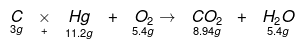Total weight g reactant = 14.2 g
= Total weight of product = 14.2 g

Q.9. The gram mole of a gas at N.T. P. occupies 22.4 L. This fact was derived from
(a) Law of gaseous volumes
(c) Berzelius hypothesis
(d) Dalton’s atomic theory

Ans. (b)
Solution.
Avogadro’s hypothesis at similar condition of temperature & pressure equal volume of different gates contains equal number of males or molecules
N2 + 3H2  ⇌ 2NH3

 Mole 1 3 2 Molecule 1 3 2 Volume 1 3 2

Q.10. Among the following pairs of compound the one that illustrates the law of multiple proportion is
(a) NH3 and NF3
(b) CO2 and CS2
(c) CS2 and FeSO
(d) SnCl2, SnCl4

Ans. (d)
Solution.
Sn + Cl2    SnCl  118 : 71
Sn + 2Cl2  → SnCl  118 : 142
Ratio = 1:2

Q.11. The percentage of carbon and oxygen in samples of CO2 obtained by different methods were found to be the same. This illustrates the law of:
(a) Conservation of mass
(b) Constant proportions
(c) Multiple proportions
(d) Reciprocal proportions

Ans. (b)
Solution.
C + O → CO2    12: 32
Sn + 2Cl2 → CaO + CO2   12: 32
2NaHCO3 →  Na2CO3 + CO2 +H2O  12 ∶ 32
Formation of a compound from different method always contain same type of element in a fixed proportion by weight.

Q.12. One of the following combinations which illustrates the law of reciprocal proportions?
(a) N2O3. N2O4, N2O5
(b) NaCl, NaBr, NaI
(c) CS2, CO2, SO2
(d) MgO, Mg(OH)2

Ans. (c)
Solution.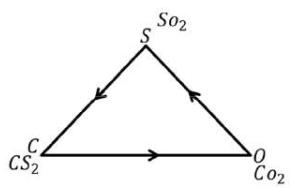Weight ratio = s/o = 32/32 + 1 : 1
Eq Ratio 8/8 = 1:1
The elements (S) & (o) combine separately with the third element (c) to form CS2 & COthey combine directly with eqach other to for SO2
CS2/CO2 = SO2 weight ratio
12/72 x 32/12 = 32/32
= 1: 1 = 1:1
Equivalent ratio:
CS2/CO2 = SO2
8 : 8 = 8 : 8
1 : 1 = 1 : 1
⇒ 12/4 × 32/4
⇒ 3:8/3:8 = 1 : 1
S : O2
⇒ 32/4 = 8
8 : 8 ⇒ 1 : 1
Equivalent ratio & weight ratio both are same it follow equivalent proportion & reciprocal propertion

Q.13. Two elements X and Y combine in gaseous state to form XY in the ratio 1: 35 .5 by mass.
The mass of Y that will be required to react with 2g of X is

(a) 7.1 g
(b) 3.55 g
(c) 71 g
(d) 35.5 g

Ans. (c)
Solution.
H + Cl → HCl
1gm reacts with 5.5 g Cl
∴ 2 gm or H reacts with = 35.5 × 2/1 = 71 gm of Cl

Q.14. Calculate the number of gram atoms in 2.3 g of sodium.
(a) 23
(b) 10
(c) 2.24
(d) 0.1

Ans. (d)
Solution.
Gram atom or mole = 2.3/2.3 = 0.1

Q.15. Calculate the mass of 2.5 gram atoms of oxygen
(a) 40 g
(b) 80.0 g
(c) 8 g
(d) 11.2 g

Ans. (a)
Solution.
2.5 gram atoms  = 2.5 mole of oxygen
1 mole (o) -- 16 gm
2.5 (o) -- 16 × 2.5
= 40 gm

Q.16. Calculate the mass of 1.5 gram molecule of sulphuric acid
(a) 151 g
(b) 129.0
(c) 147.0 g
(d) 200 gm

Ans. (c)
Solution.
1.5 gram molecule H2SO4= 1.5 mole of H2So4
1 mole of  H2SO4 -- 98 g
1.5 mole -- 98 × 1.5
= 147 g

Q.17. 19.7 kg gold was recovered from a smuggler. The atoms of gold recovered are: (Au = 197)
(a) 100
(b) 6.02 × 1023
(c) 6.02 × 1024
(d) 6.02 × 10
25
Ans. (d)
Solution.
mole  = 19.7 Kg/197 = 197 × 100/197 = 100 mole
mole×NA = total no. of atom
= 100 × 6.023 × 1023
= 6.023 × 1025

Q.18. The molecular mass of CO2 is 44 amu and Avogadro’s number is 6.02 × 1023. Therefore, the mass of one molecule of CO2 is:
(a) 7.31 × 10-23
(b) 3.65 × 10-23
(c) 1.01 × 10-23
(d) 2.01 × 10-
23
Ans. (a)
Solution.
NA molecules of Co2 = 44 gm
1 molecules = 44/6.023×10-23
= 7.31 × 10-23 gm

Q.19. What is the mass of 3.01 x 1022 molecules of ammonia?
(a) 1 kg.
(b) 0.85 g
(c) 2 gm
(d) 5 mg

Ans. (b)
Solution.
NA molecules of NH3 = 17 gm
3 × 1022 moles = 17 × 3 × 1022/6.023 × 1023
= 0.85 gm

Q.20. The largest number of molecules is in:
(a) 28 g of CO
(b) 46 g of C2H5OH
(c) 36 g of H2O
(d) 54 g of N2O
5
Ans. (c)
Solution.
(1) 28/28 = 1 mole
(2) 46/46 = 1 mole
(3) 36/18 = 2 mole
(4) 54/108 = 0.5 mole

Q.21. The number of molecules in 89.6 litre of a gas at NTP are:
(a) 6.02 × 1023
(b) 2 × 6.02 × 1023
(c) 3 × 6.02 × 1023
(d) 4 × 6.02 × 10
23
Ans. (d)
Solution.
89.6 litre of a gas ---------------?
⇒ 22.4 litre  ------- NA molecules
⇒ 89.6  litre -------  89.6 / 22.4
= 4 × 6.023 × 1023

Q.22. The density of a gaseous element is 5 times that of oxygen under similar conditions. If the molecule of the element is diatomic, what will be its atomic mass?
(a) 49.0
(b) 53.33
(c) 80
(d) 41.7

Ans. (b)
Solution.
CH4 = 6 + 4 = 10e-  present
⇒ 1.6 / 16 = 0.1 mole
Total e- =mole × NA × Total no. of e-
= 0.1 × 6.023 × 1023 × 10
= 6 × 1023 electrons

Q.23. The gram atoms present in 5 gram of Calcium are
(a) 0.125
(b) 0.21
(c) 0.117
(d) 0.512

Ans. (b)
Solution.
PV = nRT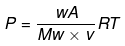P.T constant
P = d/Mw × RT  WA/V  = d
⇒ d1/d2 = Mw1/Mw2 ⇒ d/5d = 16/Mw2
Mw2 = 16 × 580

Q.24. The gram atoms present in 5 gram of Calcium are
(a) 0.125
(b) 0.21
(c) 0.117
(d) 0.512

Ans. (a)
Solution.
Gram atoms = mole
Mole = 5/40 = 0.125

Q.25. Number of moles present in 100 Kg of lime stone is
(a) 104
(b) 103
(c) 105
(d) 106
Ans. (b)
Solution.
Mole = 100 × 1023gm = 10mole
lime stone CaCo= Mw = 100 gm

Q.26. The number of molecules of CO2 in 4.4g of the gas at STP
(a) 6.02 × 1023
(b) 5.02 × 1023
(c) 6.02 × 1024
(d) 6.02 × 1022
Ans. (d)
Solution.
mole = 4.4/44 = 0.1 mole
1 mole pf Co= NA molecule
0.1 = ?
= 6.023 × 1023 ×  0.3
=  6 × 1022molecules

Q.27. Weight of 6.02 ×1020 molecules of hydrogen is
(a) 0.002g
(b) 0.02g
(c) 2g
(d) 0.01g

Ans. (a)
Solution.
6.023 × 1023
Moleculares = 2 gm of H2
6 × 1020  = 6 × 1020/6.023 × 1023

Q.28. Which of the following pairs of gases contains the same number of molecules?
(a) 11 g of CO2 and 7 g of N
(b) 44 g of CO2 and 14 g of N
(c) 22 g of COand 28 g of N
(d) All the above pairs of gases

Ans. (a)
Solution.
(a) CO2 = 11/44=0.25 × NA molecule N2 = 7/28 = 1/4 = 0.2 × NA
(b) CO2 = 44/44 = 1 × NA, 14/28 = 0.5 × NA
(c) 22/44 = 0.5 × NA,or, 28/28 × NA = 1× NA

Q.29. 2.0 × 1022 atoms of an element weights 6 g. The atomic weight of the element is approximately
(a) 290
(b) 180
(c) 34.4
(d) 104

Ans. (b)
Solution.
2 × 1022 atoms ⟶  6 g
6 × 1023  atom ----- 6 × 6 × 1023/2 × 1022
= 18 × 10
= 180 g

Q.30. A mixture of 2 mole of H2 and 1 mole of He occupies litres at NTP.
(a) 22.4
(b) 44.8
(c) 67.2
(d) 22400

Ans. (c)
Solution.
1 mole = 22.4 litre volume of any gas
2 + 1 = 44.8 + 22.4 volume
= 67.2 litre

Q.31. Which of the following will contain the same number of atoms as 20 gram of Calcium?
(a) 24 gram of Mg
(b) 12 gram of carbon
(c) 24 gram of carbon
(d) 12 gram of Mg

Ans. (d)
Solution.
20/40 = 0.5 mole
(a) 24/24 = 1 mole
(b) 12/12 = 1 mole
(c) 24/12 = 2 mole
Total no. of atoms mole × NA
= 0.5 × 6.023 × 1023

Q.32. Which of the following has the smallest number of molecules?
(a) 11.2 litre of SO2 gas at STP
(b) 1 mole of SO2 gas
(c) 1 × 1023 molecules of SO2 gas
(d) 3.2 gram of SO2 gas

Ans. (d)
Solution.
22.4 litre volume of any gas = 1 mole (stp)
(a) 11.2 litre = 0.5 mole
(b) So2 = 1  mole
(c) 6 × 1023 ----1  mole
1 × 1023 -----1023
= 1/6 mole
(d) 32/640 = 1/20 mole
Total molecular = mole × NA
=1/20 × 6.203 × 1023

Q.33. The weight of a single molecule of a substance is 8.5 × 10-23 gram. The molecular weight of the substance is
(a) 51.2
(b) 30.1
(c) 60 × 1023
(d) 14.5

Ans. (a)
Solution.

1 molecular = 8.5 × 10-23 gm
NA = ?
8.5 × 10-23 × 6.023 × 1023
= 51.2 gm

Q.34. The number of molecules present in 35.5 gram of chlorine is
(a) 3.0115 × 1015
(b) 3.0115 × 1023
(c) 2.0115 × 1023
(d) 6.023× 10
23
Ans. (b)
Solution.
35.5/35.5 = 1 × NA total atom
= 1 × NA/2 total no. of molecules
= 3 × 1023

Q.35. 16 grams of a gas at STP occupies 11.2 litres. The molecular weight of the gas is
(a) 23
(b) 25
(c) 30
(d) 32

Ans. (d)
Solution.
22.4 litre of any gas occupied mole = 1 mole
11.2 litre =16 g
22.4 litre = 16 × 22.4/11.2 = 32g

Q.36. The total number of electrons present in 18 ml of water is
(a) 6.024 × 10-24
(b) 7.240 × 10-23
(c) 6.023 × 1024
(d) 6.023 × 10
23
Ans. (c)
Solution.
Density of H2O = 1 gm/ml=1 kg/dm3
1 ml = 1 gm
Mole H2O = 18/18 = 1 mole
= 10e- = 1×10 × 6.033 × 1023
= 6 × 1024

Q.37. The ratio between the number of molecules in equal masses of nitrogen and oxygen is
(a) 7 : 8
(b) 1 : 9
(c) 9 : 1
(d) 8: 7

Ans. (d)
Solution.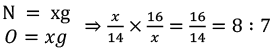⇒ 16 × NA/14 × NA = 8 ∶ 7

Q.38. Equal masses of oxygen, hydrogen and methane are kept under identical conditions.
The ratio of the volumes of the gases will be
(a) 2 : 16 : 2
(b) 2 : 16 : 1
(c) 1 : 16 : 2
(d) 1 : 1 : 1

Ans. (c)
Solution.
Equal massess
x/32 + x/2 + x/16
= (x + 16x + 2x)/32
= 1 : 16 : 2
⇒  mole ratio = volume ratio = molecular ratio

Q.39. 4.4 gram of CO2 and 2.24 litre of H2 at STP are mixed in a container. The total number of molecules present in the container will be
(a) 6.022 × 1023
(b) 1.2044 × 1023
(c) 2 moles
(d) 6.023 × 10
24
Ans. (b)
Solution.
CO2 = 4.4/4.4 = 0.1 H2 = 2.24/22.4 = 0.1
= total number of moles = 0.2
= 0.2 × 6.023 × 1023
= 1.2 × 1023

Q.40. Out of the following the largest number of atoms are contained in
(a) 11 g of CO2
(b) 4g of H2
(c) 5 g of NH3
(d) 8 g of SO
2
Ans. (b)
Solution.
(a) CO2 = 11/44 = 0.25 mole
(b) 4/2 = 2 mole
(c) 5/17 = 2 × NA largest no. of atoms
(d) 8/64 = 1/8

Q.41. The percentage of nitrogen in urea, (NH2CONH2) is:
(a) 38.4
(b) 46.6
(c) 59.1
(d) 61.3

Ans. (b)
Solution.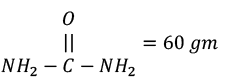⇒ 60 gm urea = 28 gN
100 g 28 × 100/60 = 46.6%

Q.42. The empirical formula of a compound is CH2O, if vapour density is 90. Then the molecular formula is
(a) CH2O
(b) C2H4O2
(c) C3H6O3
(d) C6H12O
6
Ans. (d)
Solution.
⇒ V. D × = mw
⇒ 90 × 2 = 180 gm
Empirical formula weight = 30 gm
Value of n = 180/30 = 6
C6H12O6

Q.43. An organic compound having carbon and hydrogen has 80% carbon. The empirical formula of the hydrocarbon is
(a) CH4
(b) CH3
(c) CH2
(d) CH

Ans. (b)
Solution.
C = 80%, h = 20%
%C = 80/12, %H = 20/1
= 6.66 = 20
= 6.66/6.66 = 20/6.66 = 3 {least Ratio}
1 : 3
C1H3 = CH3

Q.44. A compound contains 90% C and 10% H. The empirical formula of the compound is
(a) C8H10
(b) C15 H30
(c) C3H4
(d) C15H
32
Ans. (c)
Solution.
%C = 90/12, %H = 10/1
= 7.5/7.5 = 10/7.5 = 1.33
= 1
C3H4

Q.45. An organic compound contains C = 50% and H = 9.25%. Its empirical formula is
(a) C3H6
(b) C3H7O2
(c) C2H4O
(d) C4H8O

Ans. (b)
Solution.
C% = 50/12 = 4.1666/2.546 = 1.636 × 2
%H = 9.25%/1 = 9.25/2.546 = 2.546/2.546 = 3.633 × 2
O% = 40.76/16 = 2.546/2.546 = 1 × 2
Ch3H7O2

Q.46. A metal M having an atomic weight of 197 yields a chloride containing 35.1% chlorine.
The empirical formula of the compound is
(a) MCl
(b) MCl
(c) MCl2
(d) MCl
4
Ans. (a)
Solution.

Eq. weight of metal chloride = % of metal/% chloride × 35.5 = 64.9/35.1 × 35.5 = 64.9
Eq = 197/V
= 64.9 = 197/V= 197/64.9
VF = 3
M+3  Cl
MCL3

Q.47. When 1 gram CaCO3 is dissolved in excess dilute acid the volume of CO2 evolved at STP will be

(a) One litre
(b) 224 ml
(c) 22.4 litre
(d) 2.24 litre

Ans. (b)
Solution.
CaCO3 ⟶ Cao + CO2
1 gm  -------- 44 × 10-gm
44 g  -----  22.4 litres
44 × 10-2 g ------ = 0.224 litre = 224 ml

Q.48. 8 gram of sulphur is completely burnt in a large excess of oxygen, the volume in litres of SO2 formed as reduced to STP is
(a) 5.6
(b) 8.0
(c) 11.2
(d) 16.0

Ans. (a)
Solution.
S + O2 → SO2
32 g ------- 64 g = 1 mole = 22.4 liter
8g ----- 64 x 8/32 = 16 g
mole = 16/64 = 1/4 mole
5.6 litre value

Q.49. 0.01 mole of iodoform (CHI3) reacts with Ag powder to produce a gas whose volume at NTP i
(a) 224 ml
(b) 112 ml
(c) 336 ml
(d) 1120 ml

Ans. (b)
Solution.
2CHl3 + 6Ag ⟶ H − C ≡ C − H + 6 Agl
2 -------- 1 mole
10-2 --------- ?
10-2 × 1/2 = 0.5  × 10-2
= 5 × 10-3
∴ 1 mole ------ 22.4 literes
5 × 10-3 ------- 22.4 × 5 × 10-3
= 112 × 10-3
112 ml

Q.50. Molarity of liquid HCl with density equal to 1.17 g/ cc is
(a) 36.5
(b) 18.25
(c) 32.05
(d) 4.65

Ans. (c)
Solution.
d = M/v
1 cc = 1 ml
1000 ml = 1.17 × 1000 = 1170 g/36.5  = 32.05

Q.51. How many milliliters (mL) of 1 M H2SO4 solution are required to neutralize 10 mL of 1 M NaOH solution?
(a) 2.5 mL
(b) 5.0 mL
(c) 10.0 mL
(d) 20.0 mL

Ans. (b)
Solution.
Neutralization
Acid = Base (milliequivalent)
N1V1 = N2V2
⇒ 1 × V = 10 × 1 (N = m × VF)
V = 5ml

Q.52. The No. of moles of barium carbonate which contain 1.5 moles of oxygen atoms is
(a) 1.0 mole
(b) 1.5 mole
(c) 0.5 mole
(d) 2.0 mole

Ans. (c)
Solution.
Baco3 → 1 mole BaCo3
→ 1 mole Ba
→ 1 mole C
→ 3 mole (O)
3 mole oxygen - 1 mole BaCo3
1.5 mole oxygen - 1.5/3 = 0.5 mole BaC03

Q.53. A molecule of Haemoglobin contains 0.33% of iron by weight. The molecular weight of Haemoglobin is 67200. The number of iron atoms (At. wt. = 56) present in one molecule of Haemoglobin is
(a) 1
(b) 2
(c) 3
(d) 4

Ans.
(d)
Solution.
Mass of Fe in 1 mole of  Hb = ?
100 gm Hb – 0.33 gm Fe
1 mole(hb) = 67200g - 0.33 × 67200/100
= 221.76 gm
Mole of Fe = 221.76/56 = ≅ 3.9 ≅ 4
= 4NA atom of Fe
Na molecules of Hb – 4NA atom of Fe
1 mole - 4NA/NA
= 4 atoms of Fe

Q.54. 48 gram of Mg contains the same number of atoms as 160 gram of another element. The atomic mass of the element is
(a) 24
(b) 320
(c) 80
(d) 40

Ans. (c)
Solution.
mole of mg = 48/24 = 2 mole = 2 × NA
(A) = 160/2 = 80

Q.55. The number of moles of oxygen in one litre of air (21% oxygen by volume) at STP would be
(a) 0.186 mole
(b) 0.21 mole
(c) 2.10 mole
(d) 0.0093 mole

Ans. (d)
Solution.

In 100 ml air ---------- 21 ml oxygen
1000 ml air ----------- 21 × 1000/100 = 210 ml
22400 ml – 1 mole oxygen
210 ml - 210 × 1/22400 = 0.009375 mole oxygen

Q.56. What is correct for 10 g of CaCO3
(a) It contains 1 g atom of carbon
(b) It contains 0.3 g atoms of oxygen
(c) It contains 12 g of calcium
(d) It refers to 0.1 g equivalent of CaCO3.

Ans. (b)
Solution.
10/100 = 0.1 mole CaCO3
1 mole CaCO3 →1 mole Ca
→1 mole C
→3 mole
∴ 0.1 mole CaCO3 → 0.1 Ca
→0.1C
→0.3(oxygen)

Q.57. If isotopic distribution of C-12 and C-14 is 98% and 2% respectively, then the number of C-14 atoms in 12 g of carbon is
(a) 1.032 × 1022
(b) 3.01 × 1022
(c) 5.88 × 1023
(d) 6.02 × 10
23
Ans. (a)
Solution.
In 100 gram of carbon C-14 is=2 gram
12 gm = 2 × 12/100 = 0.24 gram
Mole of C-14 = 0.24/14 =o.017
Total no. of atoms C-14 = 0.017 × NA
= 0.0017 × 6.023 × 1023
= 0.102 × 1023
= 1.02 × 1022 atom

Q.58. The number of moles of water present in 100g of water of 90% purity is
(a) 3
(b) 18
(c) 5
(d) 5.5

Ans. (c)
Solution.
100% purity = 100/18 mole H2O
90% = 100 × 90/18 × 100
5 mole of H2O

Q.59. Which of the gases contains the same number of molecules as that of 16 grams of oxygen
(a) 16g of O3
(b) 32g of SO2
(c) 16g of SO2
(d) All

Ans. (b)
Solution.
16/16 = 1 mole Natom = NA/2 = molecals
(a) 6/48 = 1/2 × NA
(b) 32/64 = 1/2
(c) 16/4 = 1/4

Q.60. The correct arrangement of the following in order of increasing mass is
(i) N2 molecule
(ii) oxygen atom
(iii) 1 Avogram
(iv) 1 × 10-10 gram atom of copper
(a) I > II > III > IV
(b) III < II < I < IV
(c) I > III > II > IV
(d) IV > I > II > III

Ans. (d)
Solution.
(a) Nmole = 28 gm
(b) O atom = 16 gm
(c) 1/6 × 10 - 23 gm
(d) 63.5
1 mole of Cu = 63.5 gm
1 × 10-10 mol = 63.5 × 10-10 gm

Q.61. 0.5 mole of a gas (Mol. Wt. 80) occupies 11.2 litres at STP. The volume occupied by 0.25 mole of a lighter gas (Mol. Wt. 20) at STP will be
(a) 11.2 lit
(b) 5.6 lit
(c) 8 lit
(d) 22.4 litre

Ans. (b)
Solution.

0.5 mole - 11.2 litre
0.25 mole → 11.2 × 0.25/0.5 = 5.6 litre

Q.62. The molecular weight of an unknown substance is found to 24000. If it contains 0.2% Magnesium, then the number of Magnesium atoms that can be present in a molecule of it is
(a) 1
(b) 2
(c) 4
(d) 10

Ans. (b)
Solution.

100 gm-------2 gm Mg
2400--------2 × 2400/100 = 48 g
Mole of mg = 48/24 = 2 mole
Total atom of Mg = 2 × NA
NA molecules of unknown sub = 2NA
0.1 mole = 2 × NA/NA = 2

Q.63. The percentage of magnesium in chlorophyll is 2.68%. The number of magnesium atoms in 2 gram of chlorophyll is

(a) 1.34 × 1021
(b) 1.34 × 10–21
(c) 1.35 × 10–24
(d) 1.35 × 10
24
Ans. (a)
Solution.
100 g – 2.68 g Mg
2g - 2.68 × 2/100 = weight of Mg
Mole= 2.68 × 2/100 × 24 = 0.2 × 10-2
Total atom of Mg = 0.22 × 10-2 × 6.023 × 1023
= 1.34 × 1021

Q.64. Ordinary water contains one part of heavy water per 6000 parts by weight. The number of heavy water molecules present in a drop of water of volume 0.01 ml is

(a) 2.5 × 1016
(b) 5 × 1017
(c) 5 × 1016
(d) 7.5 × 10
16
Ans. (c)
Solution.
D2O = Mw = 20
Mole of D2O = 0.01/20 = 5 × 10-4 mole D2O
6000 parts ----5 × 10-4 mol D2O
1 part----- 5 × 10-4/6000 × NA
= 5 × 1016

Q.65. The number of molecules present in a drop of water. If its volume is 0.05 ml are
(a) 1.66 × 1021
(b) 1.60 × 1022
(c) 1.66 × 1023
(d) 1.60 × 10
24
Ans. (a)
Solution.
H2O density = gm/ml =1 ml=1 gm
⇒ mole H2O = 0.05/18 × NA
= 0.05/18 × 6.023 × 1023
= 1.66 × 1021

Q.66. Which of the following will not have a mass of 10 g?
(a) 0.1 mol CaCO3
(b) 1.51 × 1023 Ca2+ ions
(c) 0.16 mol of Cl-ions
(d) 7.525 × 1022 Br atom.

Ans. (c)
Solution.
(a) CaCO3 = 0.1 mol = 10g
(b) NCa2+- 408  1.51 × 1023 - 1.51 × 1023/6 × 1023 = 1/4 × 40 = 10 g
(c) 1 mole Cl =35.5  0.16 = ? 0.16 × 35.5 = 5.68 gm × 2 ≅ 10
(d) 7.525 × Br atom
1 mole – 6.023× 1023
?   ---   7.525 × 1022
⟹ 7.525 × 1022/6.2023× 1023 = 1.2 × 10-1

Q.67. x L of N2 at STP contains 3 × 1022 molecules. The number of molecules in x/2 L of ozone at STP will be
(a) 3 × 1022
(b) 1.5 × 1022
(c) 1.5 × 1021
(d) 1.5 × 10
11
Ans. (b)
Solution.
xLN2 at stp → 3 x 1022 molecules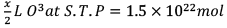∴ volume occupied by gas at S.T.P doesn’t depend on nature of gas.

Q.68. 10 ml of a gaseous hydrocarbon combustion gives 40 ml of CO2 and 50 ml of H2O vapour under the same condition. The hydrocarbon is
(a) C4H6
(b) C6H10
(c) C4H8
(d) C4H
10
Ans. (d)
Solution.
XHy + 02[x + y/4] C02 → + H2O
Volume of COformed
10x = 40ml CO2
X = 4
X = c = 4
C4H10
volume of H2O
10 y/2 = 50ml H2O
y = 100/4 = 10
y = H = 1
OR
P.O.A.C method
P.O.A.C for carbon C atom
10x = 40
X = 4
C4H10
D.O.A.C for H atom
10y/2 = 50
y = 100/10
y = 10

Q.69. 15 ml hydrocarbon requires 45 ml of O2 for complete combustion and 30 ml of CO2 is formed. The formula of the hydrocarbon is
(a) C3H6
(b) C2H6
(c) C4H10
(d) C2H
4
Ans. (d)
Solution.
CxHy + 02 → C02 + H2O
x + Y/4    x     y
(1) volume of CO2 formed
15x = 30 ml of CO2
X = 2
(2) The volume of O2 required 15x + Y/4 = 45 ml of O2
⟹ 30+15Y/4 = 45
⟹ 120+15y/4 = 45
⟹ 120+15y = 180
⟹ 15y = 180-120
15 y = 60
Y = 60/15 = 4
C2H4
x = 2   y = 4

Q.70. Complete combustion of a sample of a hydrocarbon gives 0.66 2 grams of COand 0.362 grams of H2O the formula of a compound is
(a) C3H8
(b) CH4
(c) C2H
(d) C2H
4
Ans. (a)
Solution.
hydrocarbon+ O2 → CO2 + H2O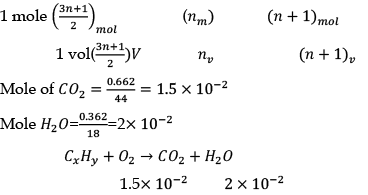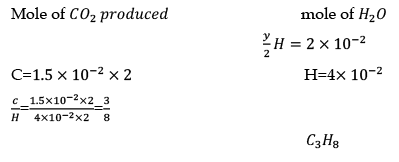Q.71. The simplest formula of a compound containing 50% of element x (atomic weight = 10) and 50% of element y (atomic weight = 20) is
(a) xy
(b) x2y
(c) xy2
(d) x2y
3
Ans. (b)
Solution.
%x = 50/10
x = 5/2.5
x = 2
y% = 50/20
y = 2.5/2,5
y = 1
x2y

Q.72. The empirical formula of an organic compound is CH. 6.023 × 1022 molecules of same organic compound weigh 7.8 g. The molecular formula is
(a) C2H2
(b) C6H6
(c) C2H4
(d) None

Ans. (b)
Solution.

empirical formula weight = 12+1 = 13g
6 × 1022− 7.8g
6 × 1023   -  ?
= 7.8 × 6 × 1023/6 × 1022
= 78 gm
n = 78/13 = 6
CnHn = C6H6

Q.73. An organic compound contains C = 21.56%, H = 4.56% and Br = 73.36%. Its molecular weight is 109. Its molecular formula is
(a) C2H5Br
(b) C3H7Br
(c) C4H8Br
(d) C6H6Br

Ans. (a)
Solution.
C% = 21.56/12 = 1.7/0.917 = 1.853 ≅ 2
H% = 4.56/1 = 4.56/0917 = 4.97 ≅ 5
Br = 73.36/80 = 0.917/0.917 = 1
n = log/log = n
e.f = m.f
= C2H5Br

Q.74. 0.078 gram of hydrocarbon occupies 22.4 ml volume at STP. The empirical formula of hydrocarbon is CH. The molecular formula of hydrocarbon is
(a) C5H5
(b) C6H6
(c) C2H2
(d) C8H
8
Ans. (b)
Solution.
22.4 ml--- 0.078 gm hydrocarbon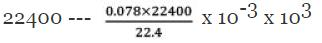= 78 g
n = 78/13 = 6      CH = 13
12+1
C6H6

Q.75. Empirical formula of a compound is CH2. The mass of one litre of this organic gas is exactly equal to that of one litre of nitrogen. Therefore the molecular formula of the organic gas is
(a) C3H8
(b) C2H6
(c) C2H4
(d) C3H
6
Ans. (c)
Solution.
e.f = CH2    12 + 2 = 14
n= 28/14 = 2
C2H4

Q.76. When 1.2 g of carbon is completely burnt in 6 litres of oxygen at STP, the remaining volume of oxygen is
(a) 3.76 lit
(b) 2.6 lit
(c) 5.8 lit
(d) 37.6 lit

Ans. (a)
Solution.
C + 1/2 O2 →  (Incomplete combustion)
1C → (1 mole oxygen)
0.1C → 0.1 × 1 = 0.1 mole of oxygen
⟹ 22.4 litre → 1 mole O2
1 C --------- 22.4 litre
0.1 ---------- 22.4 litre
6 − 2.24 = 3.76 liter

Q.77. 0.5 mole of H2SO4 is mixed with 0.2 mole of Ca (OH)2. The maximum number of moles of CaSOformed is
(a) 0.5
(b) 0.2
(c) 0.4
(d) 0.25
Ans. (b)
Solution.
H2SO4 + Ca(OH)2 →  CaSO+ 2H2O
d = 0    0.5    0.2    0
t = ?   0.5 - x   0.2 - x   x
x = 0.2
LR
L.R = 0.5/1
L.R = 0.2/1 = 0.2 = x
nx = aa
1 × = 0.2 × 100/100
x = 0.2

Q.78. 70 gram of a sample of magnesite on treatment with excess of HCl gave 11.2 litre of CO2 at STP. The percentage purify of the sample is
(a) 80
(b) 70
(c) 60
(d) 50

Ans. (d)
Solution.
Magnetic MgCO3+ Fe]=140gm
Fe + MgCO3 → MgCl2 + H2CO3

H2O = CO2
1 mole----------1CO2
1 CO2------------1 mol MgCO3
0.5--------------0.5 mol
70/140 × 100
% purity = 50%

Q.79. When 100 gram of ethylene polymerises to polythene according to the equation nCH2 = CH (CH2 - CH2)- the weight of polythene produced will be

(a) n/2 gram
(b) 100 gram
(c) 100/n gram
(d) 100n gram

Ans. (b)
Solution.

nCH2 = CH2 (-CH2 - CH2 -)n
100g    100g
n28gm -------- nx28g

Q.80. Air contains 20% by volume of oxygen. The volume of air required for the complete combustion of one litre of methane under the same conditions is
(a) 2 litre
(b) 4 litre
(c) 10 litre
(d) 0.4 litre

Ans. (c)
Solution.
CH4 +202 CO2 +2H2O
1 litre  2 litre
2 liter O2 → 1 litre CH4
20 litre ---- 20/2 = 10 litre CH4

Q.81. The hydrated Na2SO4nH2O undergoes 56% loss in weight on heating and become anhydrous. The value of n will be
(a) 5
(b) 3
(c) 7
(d) 10

Ans. (d)
Solution.

Na2SO4H2O → Na2SO4+ xH2O
142 + 18x = 56/100%
Lose in weigh due to H2O = 18x/142 + 18x = 56/100
⟹ 1800x = 142 × 56 + 18x × 56
⟹ 1800x = 7952 + 1000x
⟹ 792x = 795x
x = 10.004
x = 10

Q.82. 1.25 g of a solid dibasic acid is completely neutralized by 25 mL of 0.25 molar Ba (OH)2 solution . The molecular mass of the acid is
(a) 100
(b) 150
(c) 120
(d) 200

Ans. (d)
Solution.
Neutralisation rxn
Solid dibasic acid =
N1V1 = N2V2
1000 × w/e = N1V1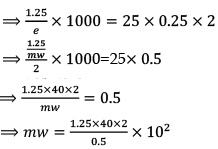mw = 160 × 1.25
mw = 200

Q.83. 0.126 g of acid requires 20 ml of 0.1 N NaOH for complete neutralization. The equivalent mass of the acid is
(a) 45
(b) 53
(c) 40
(d) 63

Ans. (d)
Solution.
w/e × 1000 = 20 × 0.1
⟹ 0.126/E × 1000 = 2
E = 0.126 × 1000/2
E = 63

Q.84. The mole fraction of the solute in one mole aqueous solution is
(a) 0.009
(b) 0.018
(c) 0.027
(d) 0.036

Ans. (b)
Solution.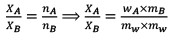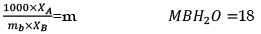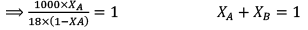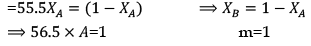XA = 1/56.5 = 0.18

Q.85. How many grams of phosphoric acid would be needed to neutralize 100 g of magnesium hydroxide?
(a) 66.7 g
(b) 252 g
(c) 112 g
(d) 168 g

Ans. (c)
Solution.

⇒ w/E1 × 1000 = w2/E2 × 1000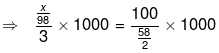⇒ 3x/98 = 200/58
⇒ x = 200 × 98 / 58 × 3 = 19600/174
= 112g

Q.86. Normality of solution of FeSO4. 7H2O containing 5.56 g / 200 mL which converts to ferric from in a reaction is  (Fe = 56, s = 32, O = 16, H = 1)
(a) 1
(b) 0.1
(c) 0.01
(d) 10

Ans. (b)
Solution.

Molarity of FeSO47H2O
156 + 126 = 278g mw
Mole = 5.56/278g mol
200-----5.56/278
1000------------?
5.56 × 1000/278 × 200 × 10-2
= 20/200 = 0.1
Fe2+ → Fe3+  VF----1
N = MXVF
N = 0.1 × 1
N = 0.1N

Q.87. 100 g of a sample of HCl solution of relative density 1.17 contains 31.2 g of HCl. What volume of this HCl solution will be required to neutralize exactly 5 litres of N/20 KOH solution?
(a) 25 ml
(b) 29.2 ml
(c) 34.2 ml
(d) 250 ml

Ans. (a)
Solution.

D = m/v
1.74 × g/m =100/V
V = 100/1.17
V = 85.4m volume of solution
⟹ 85.4 - 31.2/36.5 = 0.84
1000ml -31.2 × 1000/36.5 × 85.4
M = 10m
N= m × VF
N=10N----1
Molarity of HCl solution = 10m
Normality = molarity × VF
= 10 ×1
= 10N
N1V1 = N2V2
⟹ 10 × Vlitre  = 5/10
Vlitre = 5/100 = 0.025 litre
= 25 ml

Q.88. 300 ml of 1 M HCI and 100 ml of 1 M NaOH are mixed. The chloride ion concentration in the resulting solution is
(a) 1 M
(b) 0.5 M
(c) 0.75 M
(d) 0.25 M

Ans. (c)
Solution.
HCL ⇋ H+ + Cl-
0.3       0.3       0.3
NaOH ⇋ Na+ + OH
0.1       0.1   0.1
Cl- = 0.3/0.4 = 0.75M
Cl- = c0ncentration of Cl-1/total volume

Q.89. 200 ml of 1 M H2SO4, 300 ml 3 M HCI and 100 ml of 2 M HCI is mixed and made up to 1 litre. The proton concentration in the resulting solution is
(a) 1.25 M
(b) 1.5 M
(c) 2.5 M
(d) 0.75 M

Ans. (b)
Solution.

All are acids so it shows additive properties
H+ = (N1V1+N2V2+N3V3)/(V1+V2+V3)
400+900+200/1000 = 1500/1000 = 1.5

Offer running on EduRev: Apply code STAYHOME200 to get INR 200 off on our premium plan EduRev Infinity!

## Chemistry for JEE

223 videos|452 docs|334 tests

,

,

,

,

,

,

,

,

,

,

,

,

,

,

,

,

,

,

,

,

,

,

,

,

;